# E. Michta & G. Slade: High-dimensional near-critical percolation and the torus plateau

Abstract: We consider percolation on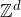at and near the critical point in high dimensions (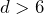for spread-out models or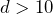for the nearest-neighbour model), where the two-point function has been proved to have power law decay at the critical point and exponential decay below the critical point. We obtain an upper bound on the two-point function that interpolates between these two regimes and which is essentially optimal. A similar result is obtained for the slightly subcritical one-arm probability. As an application, we use the near-critical decay of the two-point function to prove that throughout the critical window for percolation on a high-dimensional torus, the torus two-point function has a plateau: it decays at small distances with the same power as onbut at larger distances is essentially constant and of order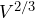where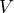is the volume of the torus. This plateau estimate leads to a new and direct proof of the torus triangle condition (which has many consequences for critical torus percolation) usingresults, without any torus lace expansion.

The main ingredients of the proof will be presented. These include previous results for high-dimensionalpercolation, the notion of pioneer edges, OSSS inequality and diagrammatic estimates.

The talk is based on joint work with Tom Hutchcroft and the two speakers.

## Video